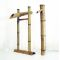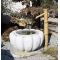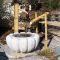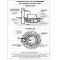##### The Japanese Bonsai specialist
Direct order Contact Help / Services Newsletter# Japanese garden fountain "shishi odoshi" 2pieces

Fontaine de bambouref. : 7237

52,00

Available quantity : 7Order

###### Description

To install a true Japanese fountain in your garden you need: A set of 2 pieces with a stationary part and a mobile part.

A big element: + - 900 mm of height + - Ø 50/60 mm, and his/her beak spout + - 230 mm of long Ø + - 20 mm.

An element to rocker of + - 650 mm of height by 340 mm of basis and a spacing of the two vertical posts of + - 185 mm.

The mobile part that topples as filling itself/themselves of water measures 400 mm of length and has a diameter of + - 50 mm.

Sold with an already gone up transparent plastic hose and ready has operate Ø 10 mm and + - 300 mm of long since the exit of bamboo. Traditional traditional manufacture. Bury under the decor a container or a piece of awning has basin that will collect the draining water. In this container place a small pump (non well stocked Pump) marks aquariophilie.qui is going to pump water in closed circuit and to carry it up in the big bamboo. Water flows out then on the small that topples, then in the container named basin "tsukubai" or "mizubachi."

On the photos some examples of bets in situation of the fountain. Non included basin. Can also be installed without basin with merely of gravels on the plastic awning.In the Japanese garden he/it murmurs of the rocker that comes to hit on a rock placed below part his/her/its resonance was supposed to move away the wolwes.

#fountain 4.8 #japanese 4.8 #garden 4.6 #bamboo 4.6 #basin 4.5 #water 4.5 #that 4.4 #part 4.4 #container 3.9 #with 3.4

Formule
(( ROUND((CHAR_LENGTH(b.article_nom)-CHAR_LENGTH(REPLACE(b.article_nom, 'japanese', '')))/LENGTH('japanese')) + ROUND((CHAR_LENGTH(b.article_description)-CHAR_LENGTH(REPLACE(b.article_description, 'japanese', '')))/LENGTH('japanese')) ) * 4.8) + (( ROUND((CHAR_LENGTH(b.article_nom)-CHAR_LENGTH(REPLACE(b.article_nom, 'fountain', '')))/LENGTH('fountain')) + ROUND((CHAR_LENGTH(b.article_description)-CHAR_LENGTH(REPLACE(b.article_description, 'fountain', '')))/LENGTH('fountain')) ) * 4.8) + (( ROUND((CHAR_LENGTH(b.article_nom)-CHAR_LENGTH(REPLACE(b.article_nom, 'garden', '')))/LENGTH('garden')) + ROUND((CHAR_LENGTH(b.article_description)-CHAR_LENGTH(REPLACE(b.article_description, 'garden', '')))/LENGTH('garden')) ) * 4.6) + (( ROUND((CHAR_LENGTH(b.article_nom)-CHAR_LENGTH(REPLACE(b.article_nom, 'basin', '')))/LENGTH('basin')) + ROUND((CHAR_LENGTH(b.article_description)-CHAR_LENGTH(REPLACE(b.article_description, 'basin', '')))/LENGTH('basin')) ) * 4.5) + (( ROUND((CHAR_LENGTH(b.article_nom)-CHAR_LENGTH(REPLACE(b.article_nom, 'water', '')))/LENGTH('water')) + ROUND((CHAR_LENGTH(b.article_description)-CHAR_LENGTH(REPLACE(b.article_description, 'water', '')))/LENGTH('water')) ) * 4.5) + (( ROUND((CHAR_LENGTH(b.article_nom)-CHAR_LENGTH(REPLACE(b.article_nom, 'part', '')))/LENGTH('part')) + ROUND((CHAR_LENGTH(b.article_description)-CHAR_LENGTH(REPLACE(b.article_description, 'part', '')))/LENGTH('part')) ) * 4.4) + (( ROUND((CHAR_LENGTH(b.article_nom)-CHAR_LENGTH(REPLACE(b.article_nom, 'that', '')))/LENGTH('that')) + ROUND((CHAR_LENGTH(b.article_description)-CHAR_LENGTH(REPLACE(b.article_description, 'that', '')))/LENGTH('that')) ) * 4.4) + (( ROUND((CHAR_LENGTH(b.article_nom)-CHAR_LENGTH(REPLACE(b.article_nom, 'container', '')))/LENGTH('container')) + ROUND((CHAR_LENGTH(b.article_description)-CHAR_LENGTH(REPLACE(b.article_description, 'container', '')))/LENGTH('container')) ) * 3.9) + (( ROUND((CHAR_LENGTH(b.article_nom)-CHAR_LENGTH(REPLACE(b.article_nom, 'pump', '')))/LENGTH('pump')) + ROUND((CHAR_LENGTH(b.article_description)-CHAR_LENGTH(REPLACE(b.article_description, 'pump', '')))/LENGTH('pump')) ) * 3.4) + (( ROUND((CHAR_LENGTH(b.article_nom)-CHAR_LENGTH(REPLACE(b.article_nom, 'with', '')))/LENGTH('with')) + ROUND((CHAR_LENGTH(b.article_description)-CHAR_LENGTH(REPLACE(b.article_description, 'with', '')))/LENGTH('with')) ) * 3.4)

## Secure payment## Delivery

Our logistic partners :